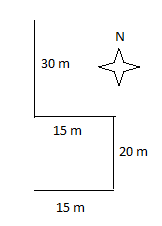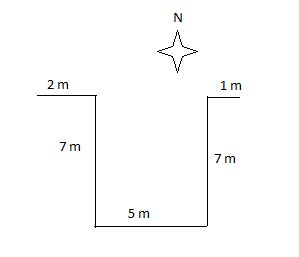0
182

Here you can download TISSNET 2022 Time, Speed & Distance [PDF] by Cracku. Very Important Time Speed Distance Questions for TISSNET 2022 based on asked questions in previous exam papers. These questions will help your TISSNET exam preparation. So kindly download the PDF for reference and do more practice.

Question 1: A man can row 10.2 km downstream in 18 minutes. If the speed of the stream is 3.5 km/h, how much time he would take to cover 121.5 km upstream? (in hours)

a) $4\frac{1}{2}$

b) $3$

c) $4$

d) $5\frac{1}{2}$

e) $3 \frac{1}{2}$

Question 2: Mohan walked 30 metres towards South took a left turn and walked 15 meters.He then took a right turn and walked 20 meters He again took a right turn and walked 15 meters How far is he from the starting point ?

a) 95 meters

b) 50 meters

c) 70 meters

d) Cannot be determined

e) None of these

Question 3: A car covers the first 35 km of its journey in 45 minutes and covers the remaining 69 km in 75 minutes. What is the average speed of the car?

a) 42 km/hr

b) 50 km/hr

c) 52 km/hr

d) 60 km/hr

e) None of these

Question 4: A man riding a bicycle completes one lap of a circular field along its circumference at the speed of 79.2km/h in 2 min 40 s What is the area of the field ?

a) $985600 m^{2}$

b) $848500 m^{2}$

c) $795600 m^{2}$

d) Cannot be determined

e) None of these

Question 5: A 240 m long train crosses a platform twice its length in 2 min what is the speed of the train ?

a) 8 m/s

b) 4 m/s

c) 6 m/s

d) Cannot be determined

e) None of these

Question 6: Riya Manasvi and Pintu start running around a circular stadium and complete one round in 24 s, 6 s and 14 s respectively. In how much time will they meet again at the starting point ?

a) 1 min 32 s

b) 4 min 8 s

c) 3 min 25 s

d) 2 min 48 s

e) None of these

Question 7: Parag walks 226 m everyday How many Kilometers will he walk in five weeks ?

a) 6.57

b) 7.91

c) 8.23

d) 9.41

e) None of these

Question 8: A truck covers a distance of 360 Km in 8 h. A car covers the same distance in 6 h What is the respective ratio between the speed of the truck and the car ?

a) 3:5

b) 3:4

c) 1:2

d) 4:5

e) None of these

Question 9: Ajay walked 2m towards east, took a right turn and walked 7m. He then took a left turn and walked 5m before taking a left turn and walking 7m. He then took a final right turn and walked 1 m before stopping. How far is Ajay from the starting point?

a) 8m

b) 7m

c) 6m

d) 5m

e) 9m

Question 10: A 200 meter long train crosses a platform double its length in 36 seconds What is the speed of train in kmph ?

a) 60

b) 48

c) 64

d) 66

e) None of these

Let the speed of boat rowed by man by x.
A man can row 10.2 km downstream in 18 minutes i.e speed (x+3.5) = (10.2*60)/18
Hence, speed of boat = 30.5 km/hr
Now, relative speed of boat going downwards = 30.5-3.5 = 27km/hr
Now, number of hours required to row upwards = 121.5/27 = 4.5 hours = 4$\frac{1}{2}$
Option A is correct.

The data can be intrepreted in diagrammatic form as:Hence, his distance from the starting point = 30+20 = 50 m
Option B is correct.

Average speed = Total distance / Total time taken

Total distance = 35 + 69 = 104 km

Total time = 45 + 75 = 120 min = 2 hr

Average speed = $\frac{104}{2} = 52~km/hr$

Circumference of circular field = Distance covered by cyclist in one lap = (79.2*1000)/3600 * 160 = 3520 m
Area of the field = $985600 m^{2}$
Therefore, option A is correct.

The length of platform = 2*length of train = 2*240 = 480
Total distance covered by train = Length of platform + Length of train = 480+240 = 720
Speed of train = 720/120 = 6m/s
Hence, the correct option is option C.

The time of their meeting again can be calciulated by taking LCM of 24, 6 and 14.
LCM of 24, 6 and 14 = 168
Now, 168 seconds = 2 min 48 s.
Option D is correct.

Number of days in 5 weeks = 5*7 = 35 days
Number of kilometres wallked in one day = 0.226 kilometres
Number of kilometres walked in 35 days = 0.226 * 35 = 7.91 km
Hence, option B is the correct answer.

Speed of the truck = 360/8 = 45 km/hr
Now,
Speed of the car = 360/6 = 60km/hr
Ratio of speed of truck and car = 45/60 = 3:4
Therefore, option B is correct.